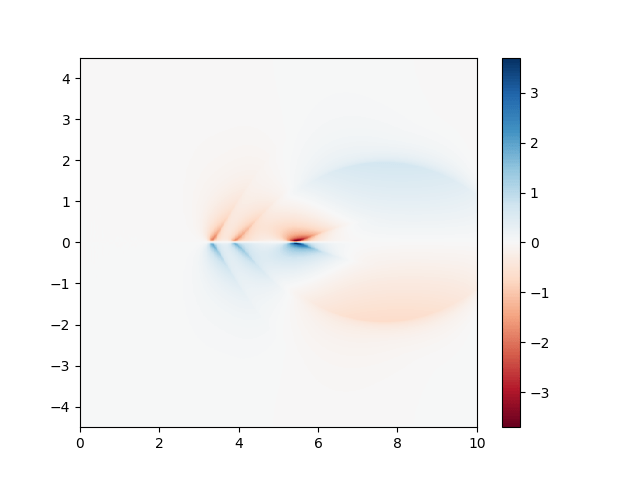# #StackBounty: #python #neural-network #convolutional-neural-network #overfitting Is it possible to use a Neural Network to interpolate …

### Bounty: 50

I am completely new to Artificial intelligence and Neural Networks. I am currently working on a plasma physics simulation project which requires a very high resolution data set. We currently have the results of two simulations of the same problem run at different resolutions – with one’s resolution being higher than the other. However, we need an even higher resolution for us to use this data effectively. Unfortunately, it is not possible for us to run a higher resolution simulation because of computation power limitations. So instead, we are trying to somehow interpolate the data we have to get a reasonable estimate of what the simulation result might be if we were to run it at a higher resolution. I tried to interpolate the data using conventional interpolation techniques and functions in SciPy. However, the interpolated result is sometimes off by about 20 to 30 percent at certain points.

Problem Statement and my Idea

So I was wondering if it was possible to use a neural network to generate an output that when fed into the interpolator (code that I have written using SciPy), would yield better results than if I used just the interpolator. Currently, out data when plotted looks like this:This is the data plotted at a certain time t. However, we have data similar to this for about 30 different times steps – so we have 30 different data sets that look similar to this but are slightly altered. And as I said before, we also have the high resolution and low resolution data sets for each of the 30 timesteps.

My idea for the ANN is as follows: The low resolution data (a 512 x 256 2-D array) can be fed into a the network to output a slightly modified 512 x 256 2-D array. We can then input this modified data set into our interpolator and see if it matches the high resolution data set (1024 x 512). The error function for the network would be a function of the difference of the high data set and the interpolated data set (maybe something like the sum of the squares of the difference of each element in the arrays). This can then be done for all 30 different data sets to minimise the difference in the high res and interpolated data sets.

If this works as planned, I would somehow use this trained ANN to the high resolution data set (1024 x 512) to feed it’s output into the interpolator.

Questions

Is it possible to create a neural network that can do this, and if yes, what type of networks do this?

Even if the neural network can be trained, how do we upgrade to work for the high res data set (1024 x 512) when it was initially trained with the low res data set (512 x 256)?

Is this a trustworthy method to predict simulation results? (All 30 data sets look almost exactly like the image above; including the high res results)